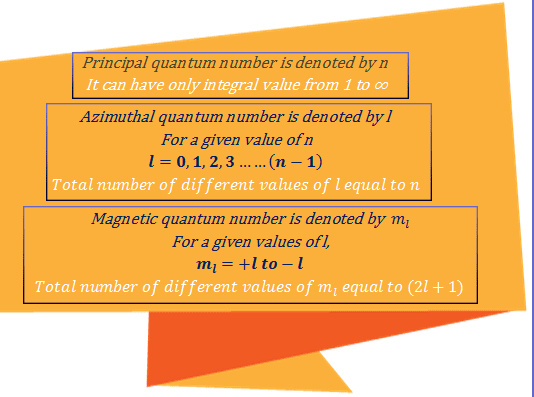# Atomic theory questions and answers

### Bohr's atomic theory

According to Rutherford's atomic theory, the entire mass of an atom was concentrated in a tiny positively charged nucleus around which the extranuclear electrons are moving in constant motion in the orbit.
But a moving charged particle emits radiation, will then loss kinetic energy and eventually hit the nucleus.
To resolve the anomalous position Bohr's atomic theory was proposed which is based on several novel postulates.
Following formulas will be widely used in solving the questions of Bohr's atomic theory.Atomic theory questions and answers
• Question
The energy of an electron in the first Bohr orbit of the hydrogen atom is -13.6 eV. What is the energy value of the electron in the excited state of Li⁺²?
• Answer
The energy of an electron in nth orbit, En = (E₁/n²) × Z²
For Li⁺², Z = 3 and in the excited state of Li⁺², n = 2.
Putting the values on the above equation we have,
ELi⁺² = (-13.6/4) × 3²
= - 30.6 eV
• Question
What is the radius of the second orbit of a hydrogen atom? Give radius of first orbit = 0.529 Å.
• Answer
The radius of an electron in nth orbit, rn = r₁ × n²
For second orbit n = 2.
Thus the radius of the second orbit of a hydrogen atom,
r₂ = 0.529 × 2²
= 2.12 Å

### Quantum number orbitals

For size shape and orientation of an orbital, we need to know about the four quantum numbers and these quantum numbers are designed as, principal quantum number(n), azimuthal quantum number(l), magnetic quantum number(m) and spin quantum number(s).
Following rules will be widely used in solving the questions of quantum number orbitals.Quantum numbers
• Question
Write the correct set of four quantum numbers for the valence electron of Rubidium.
• Answer
The atomic number of Rubidium atom is 37. Thus the electronic configuration of rubidium is,
1S² 2S² 2P⁶ 3S² 3P⁶ 4S² 3d¹⁰4P⁶5S¹
or, [Kr]³⁶ 5S¹
So the valence electron of rubidium means 5S¹ electron of a rubidium atom. Thus for 5S¹ electron, principal quantum number = 5, azimuthal quantum number = 0, magnetic quantum number = 0, and spin quantum number = +½.
So the correct set of four quantum numbers for the valence electron of Rubidium atom are,
5, 0, 0, +½
• Question
What are the four quantum numbers of the 19th electron of Cr atom?
• Answer
The four quantum numbers of the 19th electron of Cr atom are 4, 0, 0, +½
• Question
How many electrons in an atom can have the following quantum numbers n=4 and l=1?
• Answer
n = 4 means principal quantum number 4 and l = 1 means the azimuthal quantum number is 1, that is P orbital.
Thus when n =1 and l = 0 we can describe 4P orbital. For P orbital magnetic quantum numbers = -1, 0, +1 and each magnetic quantum number has two spin quantum numbers.
So (2 × 3) = 6 electrons in an atom can have the following quantum numbers n =4 and l =1.
• Question
How many possible orbitals are there for n = 4?
• Answer
n = 4 means principal quantum number 4.
Thus when n =4, l = 0, 1, 2, 3. That is 4S, 4P, 4d, and 4f orbitals. S orbital has one orientation of space, p orbitals have three orientation in space, d orbitals have five orientation in space, and f orbitals have seven orientation in space.
Thus the number of possible orbitals when n = 4 is (1 + 3 + 5 + 7) = 16
• Question
How many possible orbitals are there for n = 3, l =1, and ml =0 ?
• Answer
n = 3 means principal quantum number 3, l = 1 means azimuthal quantum number = 0, and ml = 0 means magnetic quantum number zero that is one orientation in space.
Thus the number of orbitals is 1, 3S orbital.

Atomic theory questions and answers related to Bohr's atomic theory and quantum number and orbitals

This is the most recent post.
Older Post

## Inorganic Chemistry

[Inorganic chemistry][column1]
[blogger]

Name

Email *

Message *